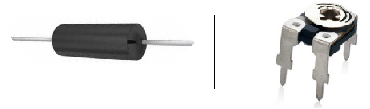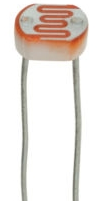﻿ Types of Variable Resistors# Types of Variable ResistorsVariable Resistors are resistors whose resistance values can vary according to some factor applied to them. For example, potentiometers are variable resistors that changes when a user adjusts the knob. Photoresistors are variable resistors which change according to the light that strikes its surface.

In this article, we will go over the many various types of variable resistors that exists and explain how they work and operate in circuits.

### Types of Variable Resistors

#### PotentiometersA potentiometer is a 3-terminal variable resistor. By adjusting the wiper terminal, it can be used to represent a wide range of resistances in a circuit from anywhere near 0Ω to the specified resistance rating of the potentiometer. Therefore, for example, a 10KΩ potentiometer can be adjusted to give the resistance range from almost 0Ω to 10KΩ by adjusting the potentiometer knob.

Potentiometers are in wide use in circuits for a variety of uses, but their main fuction remains the same: to increase or decrease the gain of a signal in a circuit. When the resistance of the potentiometer is decreased, the gain of the signal increases. When the resistance is increased, the gain of the signal decreases. This can be used in circuits to control volume levels such as on speakers or for any adjustable controls.

#### RheostatsA rheostat is a 2-terminal variable resistor.

As a variable resistor, it serves to vary the amount of voltage or current in a circuit.

Just like potentiometers, rheostats can be used to vary AC or DC signals. They differ from potentiometers in the fact that they only have two leads of which potentiometers have 3. However, the two still achieve the same function. While a potentiometer uses a third terminal to serve as the adjustable part, the rheostat uses a slider to vary resistance. If the slider isn't moved, the rheostat functions as a fixed resistor.

A rheostat is connected in a circuit by having the two leads connected in series with the load.

#### ThermistorsA thermistor is a thermally sensitive resistor whose resistance value changes with changes in operating temperature.

Because of the self-heating effect of current in a thermistor, the device changes resistance with changes in current.

Thermistors exhibit either a positive temperature coefficient (PTC) or a negative temperature coefficient (NTC). If a thermistor has a positive temperature coefficient, its resistance increases as the operating temperature increases. Conversely, if a thermistor has a negative temperature coefficient, its resistance decreases as the operating temperature increases.

How much the resistance changes with changes in the operating temperature depends on the size and construction of the thermistor. It's always best to check the datasheet of the thermistor in use to find out all the specifications of the thermistors.

Thermistors are frequently used in electronic circuits that handle temperature measurement, temperature control, and temperature compensation.

#### PhotoresistorsPhotoresistors are resistors whose resistance values change according to the light striking the surface of the resistor. In a dark environment, the resistance of a photoresistor is very high, possibly several MΩ, depending on the resistance rating of the specific photoresistor in use. When intense light hits the surface, the resistance of the photoresistor drops dramatically, possibly to as low as 400Ω.

Thus, photoresistors are variable resistors whose resistance values change in regard to the amount of light hitting its surface.

The above list is an extensive list of the the types of variable resistors that exist today. Engineer designers choose the type of variable resistance needed in a circuit with the element they want the resistance to be changed by.

Related Resources